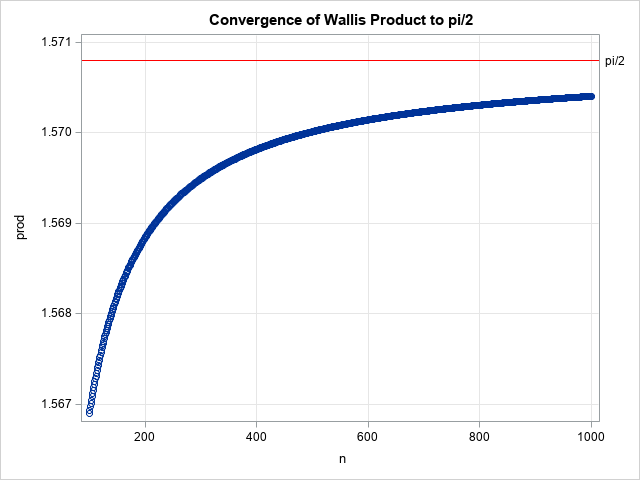This is my Pi Day post for 2021. Every year on March 14th (written 3/14 in the US), geeky mathematicians and their friends celebrate "all things pi-related" because 3.14 is the three-decimal approximation to pi. Most years I write about lower-case pi (π), which is the ratio of a circle's circumference to its diameter. But this year I thought it would be fun to write about upper-case pi ($\Pi$), which is the mathematical notation for a product of terms.

Just as $\Sigma$ is used to express a summation, so $\Pi$ is used to express a product. Did you know that there are infinite products that converge to pi? A famous one is the Wallis product, which converges to π/2:
$\pi / 2 = \prod_{n=1}^{\infty} \left( \frac{2n}{2n-1} \cdot \frac{2n}{2n+1} \right) = \left( \frac{2}{1}\cdot \frac{2}{3} \right) \cdot \left( \frac{4}{3}\cdot \frac{4}{5} \right) \cdot \left( \frac{6}{5}\cdot \frac{6}{7} \right) \cdot \left( \frac{8}{7}\cdot \frac{8}{9} \right) \cdot \;\cdots$

### Implement Wallis's formula for pi

Suppose you wanted to implement Wallis's formula on a computer. How would you do it? The simplest way is to literally translate the formula into a loop that multiplies the first N terms in the product. You can plot the partial products against the number of terms to see how quickly (or slowly!) the product converges, as shown in the following SAS DATA step:

ods graphics / reset; %let N = 1000; data WallisProd; prod = 1; do n = 1 to &N; term = (2*n/(2*n-1)) * (2*n/(2*n+1)); prod = prod * term; output; end; run;   title "Convergence of Wallis Product to pi/2"; proc sgplot data=WallisProd; where n >= 100; scatter x=n y=prod; refline &pi2 / axis=y noclip lineattrs=(color=red) label="pi/2"; xaxis grid; yaxis grid; run;The graph indicates that the convergence of the Wallis product is very slow. In fact, you can prove that the n_th term of the product has an asymptotic error of size π/(8n) as n → ∞. Because of this, the Wallis formula is not a good choice for computing the digits of pi. It takes about 400,000 terms to get six-digit accuracy!

But let's not focus on π, let's focus on the product, $\Pi$. Although a direct translation of the product formula is simple, it is not necessarily the best way to compute the product, computationally speaking. Pi Day seems like a great time to review an alternative computational method that is often useful when you compute with products.

### Products and log transformations in statistics

The Wallis product is well behaved because the individual terms are all close to 1. However, many products that you encounter in computational statistics are not so well behaved. For example, in statistical modeling, you encounter the likelihood function. Given a set of data (x1, x2,..., xn) and a probability density function (f) that you believe models the data, the likelihood of the data is the product $\prod_{i=1}^{n} f(x_{i})$. In this formulation, every term in the product is less than 1. If the data contain outliers, there might be individual probabilities that are tiny. Therefore, a naive computation of the product can result in a very small number. In fact, the computation might encounter an underflow error, especially for large data sets.

A computational technique that avoids this potential problem is to apply a log transformation, which converts the product into a summation of the log of the terms. After you have computed the sum, you exponentiate it to obtain the product. This technique requires that all terms in the product be positive, which is true in most applications.

In symbols, if you have a product of positive values, $y = \prod_{i} a_{i}$, you can log-transform it to $\log(y) = \sum_{i} \log(a_{i})$. The product is mathematically equivalent to $y = \exp\left( \sum_{i} \log(a_{i}) \right)$.

Let's apply the log transformation to the Wallis formula for π/2. The following SAS DATA step computes a sum of log-transformed terms. After each step, the EXP function gives the value of the product at that step.

data WallisSum; do n = 1 to &N; logterm = 2*log(2*n) - log(2*n-1) - log(2*n+1); /* log transform of terms */ logprod + logterm; /* summation */ prod = exp(logprod); /* exp(sum) */ output; end; run;

The graph of the partial products is the same graph as in the previous section. The numerical difference between the two computations is less than 3E-14. However, in general, it is usually safer to sum the log-transformed terms because the method avoids numerical overflow and underflow issues that are common when you compute a product in a naive way.

So, this Pi Day, don't just celebrate lower-case pi, π = 3.14159265.... Take a moment to think about upper-case pi ($\Pi$), which stands for a product of numbers. For many products, a numerically stable way to compute the product to log-transform the problem, compute a summation, and then exponentiate the sum. And that is a numerical technique that is worth celebrating, not just on Pi Day but every day!